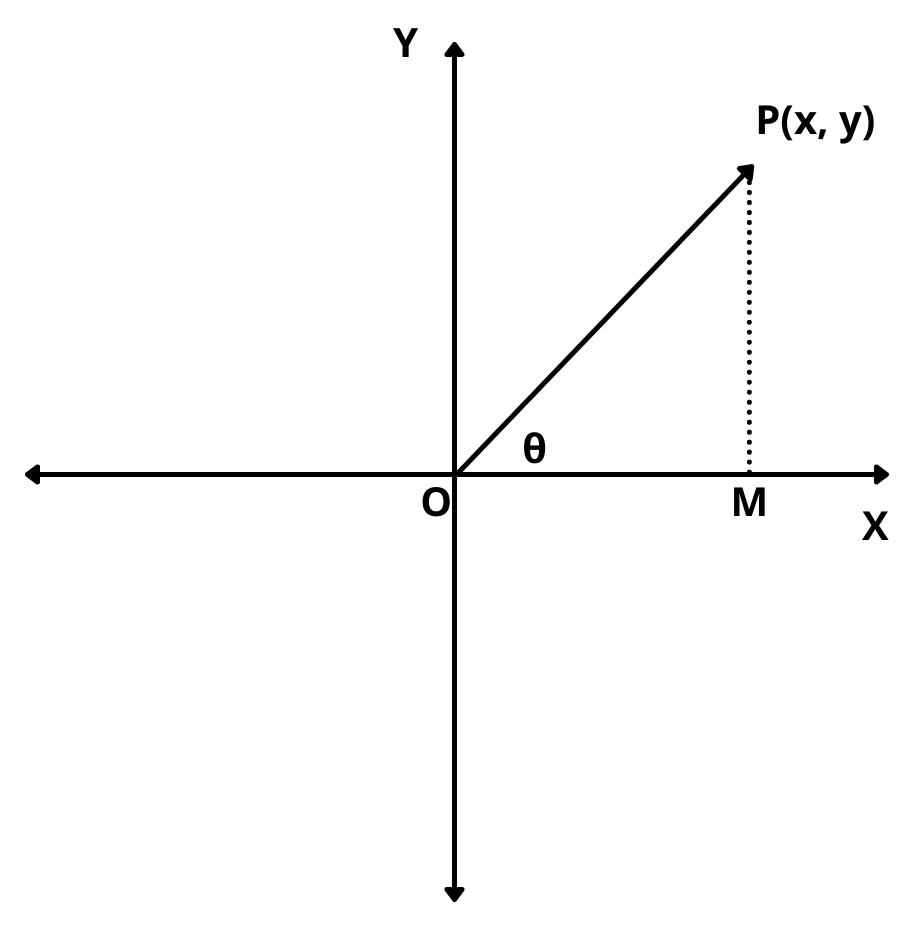# Polar Form of Complex Numbers with Examples

Here you will learn what is the polar form of complex numbers with examples.

Let’s begin –

## Polar Form of Complex Numbers

Let z = x + iy be a complex number represented by a point P(x, y) in the argand plane.Then by the geometrical representation of z = x + iy, we have

OP = | z |  and angle POX = $$\theta$$ = arg (z)

In triangle POM, we have

$$cos\theta$$ = $$OM\over OP$$ = $$x\over | z |$$ $$\implies$$ x = | z | $$cos\theta$$

and, $$sin\theta$$ = $$PM\over OP$$ = $$y\over | z |$$ $$\implies$$ x = | z | $$sin\theta$$

So,  z = x + iy

z = | z | $$cos\theta$$ + i | z | $$sin\theta$$

$$\implies$$  z = | z | $$(cos\theta + i sin\theta)$$

$$\implies$$ z = r$$(cos\theta + i sin\theta)$$, where r = | z | and $$\theta$$ = arg (z)

This form of z is called a polar form of z. If we use the general value of the argument of $$\theta$$, then the polar form of z is given by

z = r$$(cos(2n\pi + \theta) + i sin(2n\pi + \theta))$$, where r = | z | and $$\theta$$ = arg (z) and n is an integer.

Example : Write the following complex numbers in the polar form :

(i) $$-3\sqrt{2} + 3\sqrt{2}i$$

(ii) 1 + i

Solution :

(i) Let z = $$-3\sqrt{2} + 3\sqrt{2}i$$. Then,

r = | z | = $$\sqrt{(-3\sqrt{2})^2 + (3\sqrt{2})^2}$$ = 6

Let $$tan\alpha$$ = |$$Im(z)\over Re(z)$$| = 1 $$\implies$$ $$\alpha$$ = $$\pi\over 4$$

Since the point representing z lies in the second quadrant. Therefore, the argument of z is given by

$$\theta$$ = $$\pi – \alpha$$ = $$\pi$$ – $$\pi\over 4$$ = $$3\pi\over 4$$.

So, the polar form of z is

z = r$$(cos\theta + i sin\theta)$$ = 6$$cos{3\pi\over 4} + isin{3\pi\over 4}$$

(ii) Let z = 1 + i. Then,

r = | z | = $$\sqrt{1^2 + 1^2}$$ = $$\sqrt{2}$$

Let $$tan\alpha$$ = |$$Im(z)\over Re(z)$$| = 1 $$\implies$$ $$\alpha$$ = $$\pi\over 4$$

Since the point (1, 1) representing z lies in the first quadrant. Therefore, the argument of z is given by

$$\theta$$ = $$\alpha$$ = $$\pi\over 4$$

So, the polar form of z is

z = r$$(cos\theta + i sin\theta)$$ = $$\sqrt{2}$$$$cos{\pi\over 4} + isin{\pi\over 4}$$